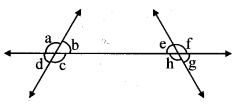# Maharashtra Board 8th Class Maths Practice Set 2.1 Solutions Chapter 2 Parallel Lines and Transversals

Balbharti Maharashtra State Board Class 8 Maths Solutions covers the Practice Set 2.1 8th Std Maths Answers Solutions Chapter 2 Parallel Lines and Transversals.

## Practice Set 2.1 8th Std Maths Answers Chapter 2 Parallel Lines and Transversals

Question 1.
In the given figure, each angle is shown by a letter. Fill in the boxes with the help of the figureCorresponding angles:
i. ∠p and __
ii. ∠q and __
iii. ∠r and __
iv. ∠s and __

Interior alternate angles:
v. ∠s and __
vi. ∠w and __
Solution:
i. ∠w
ii. ∠x
iii. ∠y
iv. ∠z
v. ∠x
vi. ∠r

Question 2.
Observe the angles shown in the figure and write the following pair of angles.1. Interior alternate angles
2. Corresponding angles
3. Interior angles

Solution:

1. ∠c and ∠e; ∠b and ∠h
2. ∠a and ∠e; ∠b and ∠f; ∠c and ∠g; ∠d and ∠h
3. ∠c and ∠h; ∠b and ∠e